# Statistical Gaslighting 

Every Monday, the previous week is statistically concluded with the announcement of the number of positive PCR tests from Sunday. On 01.02.2021  and 08.02.2021 , RTL published an article on this. Each time, the article was titled with the statement that the infection figures had increased by 19% and 14% respectively. The second article shows the following graph:

The increase therefore refers to the percentage change (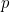) in the absolute weekly numbers (or the corresponding mean values) from the previous week . It is calculated as follows: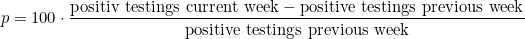Let’s put the numbers in context and add some information to the table.

The more you test, the more positives you will find. It is therefore essential to look not at the absolute number but at the proportion of the total number of positive tests (positive rate).

The number of tests increased by around 13 and 20 per cent in calendar weeks 4 and 5 respectively.

The 7-day incidence, which is not listed in the table, is calculated directly from the number of positive tests in one week. For the current population of 626108 people applies: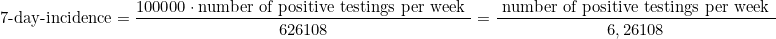It thus differs only by a factor from the absolute number of positive tests and thus varies in the same way. The example of week 5 also shows here that an increase in the 7-day incidence in no way indicates a worsening of the epidemiological situation.

The following graphs visualise the data of the table once again: total number of tests (blue), positive tests (red), positive rate (green) and 7-day incidence (dark red).

It remains to mention that for calendar week 6, with 1099 test positives and a total of 70608 tests, the absolute number fell by 41, which corresponds to a percentage change of -3.6%. In relation to the positive rate of 1.56%, this is even a percentage change of around -16%.

### Sensitivity and specificity

From a scientific point of view, the testing of individuals in a given period of time represents a measurement for which it is not usual to publish the result without an error analysis. Diagnostic tests in general, and thus the PCR test used for the detection of the SARS-CoV-2 virus in particular, always have a certain margin of error.

We have already briefly dealt with the characteristic properties of a test in our article on PCR tests . Since they play a decisive role in the calculation of the error rate, we would like to go into the mathematical basics of this a little more deeply.

Depending on whether one has a disease or not, and depending on whether the corresponding diagnostic test is positive or negative, there are 4 possible situations, which are shown in the following table.

To evaluate the reliability of a test, one looks at the two properties sensitivity and specificity :

The sensitivity indicates how reliably a person with the disease is recognised as such. A test with a sensitivity of 100% therefore detects all patients correctly: the proportion of true positives (RP) is correspondingly 100%, and of false negatives (FN) 0%. Lower sensitivities reduce the proportion of RP and increase the proportion of FN.

Specificity is responsible for the recognition of healthy persons: how reliably is a healthy person recognised as such? A specificity of 100% correctly identifies all healthy people: the proportion of correct negatives (RN) is 100%, false positives (FP) are non-existent. Accordingly, a lower specificity increases the proportion of FP and decreases the proportion of RN.

The number of people who are actually ill is therefore calculated from the sum of RP and FN (top row in the table) and does not correspond to the number of positive tests. If we look at the proportion of the total number of people tested, we get the prevalence. It should be noted that the prevalence is generally not known a priori and must be estimated!

Since no test can demonstrate a sensitivity and specificity of 100%, it is a mathematical fact (Bayes’ theorem) that if only a small proportion are ill, hence the prevalence is low, the proportion of FP increases in terms of positive tests .

Instead of explaining the mathematical basics of Bayes’ theorem, we show its effect in two examples, one with high and one with low prevalence.

Example 1: Let us assume a prevalence of 30%, a sensitivity of 99% and a specificity of 98%. The proportions in the table are then calculated:

The number of people who tested positive (31.1%) is a good value for the 30% who are actually ill; the proportion of negative tests (68.9%) also corresponds well with the actual (70%). The proportions of FP and FN are low in this case. The probability that someone who tests positive is actually ill is therefore high. This is called the positive predictive value (PPV). It is calculated from the quotient: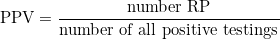Here, the result for theis therefore: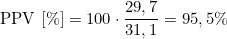In other words, the probability that someone who tests positive is really sick is 95.5%.

Example 2: The prevalence is now 1%, sensitivity and specificity remain the same. The result for the table is:

In a test with these specifications, 2.97% will therefore receive a positive test result, but only 1% are actually ill, i.e. just under a third. In this case, the proportion of FP is twice as high as that of RP. The probability that someone who tests positive is actually ill is small.

For the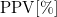applies accordingly:The proportion of those tested negative (97.03%), however, differs only insignificantly from that of those actually healthy (99%).

One more essential point in conclusion: For the share of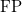applies:In the case that no one is ill (prevalence = 0%), the proportion ofis still. This proportion of positive tests represents the minimum that cannot be undercut.

Example: With a specificity of 99%, there will always be at least 1% positive tests, regardless of prevalence!

It should be noted in this context that exact values for sensitivity and specificity for the tests used in Luxembourg were not officially communicated for a long time. It was only in response to a parliamentary question on 9 July 2020  that Ms Lenert replied that the specificity would be between 99 and 100% and that it would therefore not be necessary to retest for confirmation in the case of a positive test. A value for the sensitivity was not given. According to our information, the indication of 20% false negatives refers to severe courses with typical symptoms, where the patients were nevertheless tested negative  and can therefore not be transferred to the general test situation.

### It’s all in the mix

The daily communicated results of the PCR tests refer to all tests performed on the previous day, or possibly earlier. Currently, however, testing is known to take place in two very different contexts.

One is the Large Scale Test, to which the test station at the airport can also be counted. It is significant here that there is no suspicion of infection in the persons tested. The positive rate is correspondingly low.

The other tests are carried out on a doctor’s prescription (“ordonnance”). This is the case if a person is symptomatic. In the period considered here, tracing, i.e. the follow-up and verification of persons who were in contact with a person who tested positive, was also assigned to this category. As expected, the positive rate is higher here.

The percentage breakdown is shown for calendar weeks 2020/43 to 2021/5 in the following chart:

The global positive rate includes all tests of the respective week and is directly related to the positive rates of “ordonnance” and LST via the weighted mean .

Example: In calendar week 5, the ratio was “ordonnance” to LST, 34.33% to 65.6%. The positive rates were 4.83% and 0.3% respectively. The global positive rate then results in: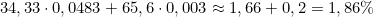The course of the 3 positive rates for calendar weeks 2020/44 to 2021/5:

Let us first look at the positive rate of the LST: from week 52 it fluctuates by 0.3%. If we assume that this is the minimum, we can conclude that these are almost exclusively false positives, which therefore corresponds to a specificity of approx. 99.7%.

The absolute number of people tested positive for LST in week 5, for example, was 121, which corresponds to 0.3%. These should therefore not actually be taken into account in the statistics, or at least reference should be made to the fact that a significant error can occur here due to the very low prevalence.

In the „prescrption” group, the positive rate is of course higher. In week 5 we had 1019 positives to 21109, which corresponds to a positive rate of 4.83%. Since this is therefore almost a factor of 15 greater than in the LST group, it essentially determines the global positive rate. The same applies alone to the distribution of the shares of “ordonnance” and LST in the total number of tests.

If we compare the positive rates in the two categories, it should be clear that looking only at the global positive rate distorts the actual situation. After all, the virus is virtually non-existent in the general population, and therefore only prevalent in a relatively small group. Even here, only every twentieth person tests positive, and in addition, contrary to a current WHO guideline , those who are asymptomatic are not tested.

### Closing Words

It should be obvious to everyone that reality simply cannot be defined with a few numbers, and yet we currently seem to settle for that for most of the time.

Which, however, is unacceptable is the continuous communication of absolute numbers that are not put into a meaningful context and in this way cannot be put into perspective. Furthermore, the fact that “negative” developments usually receive broad media attention, while “hopeful” evolutions are usually hushed up, may suggest that objective reporting is not always the aim.

There seems to be no awareness of the necessity, or even knowledge, that every scientific measurement must of course be checked for reliability. One confines oneself to passing numbers (actually “raw data” in the literal sense) and suggests to the public that these data are reliable and significant.

We hope to have illustrated with this article that the situation itself is much more complex and also requires a correspondingly elaborate examination. Moreover, we have only looked at the statistical aspect. Before the Covid19 pandemic, it would have been unthinkable in medicine that a diagnostic test alone would be (capable of and) sufficient to assess the health status of a person.

### Sources:

1] In psychology, gaslighting […] is a form of psychological violence or abuse by which victims are deliberately disoriented, manipulated and deeply unsettled, and their sense of reality and self is gradually deformed or destroyed. Source: Wikipedia https://de.wikipedia.org/wiki/Gaslighting

 RTL (01.02.2021): Infection rate increased by 19% within one week https://www.rtl.lu/news/national/a/1664746.html

 RTL (08.02.2021): Infection rate increased by 14% within one week https://www.rtl.lu/news/national/a/1668631.html

 maths2mind: Modificaton dimensions https://www.maths2mind.com/schluesselwoerter/relative-anderung

 Expressis Verbis: PCR-Test
https://www.expressis-verbis.lu/2021/02/11/pcr-test/

 Lecturio: Epidemiology: Statistical basics https://www.lecturio.de/magazin/epidemiologie-statistische-grundlagen/

7] Institute of General Practice and Health Services Research: On the Necessity of a Methodology of Graded Diagnostics in General Practice as a Consequence of Bayes’ Theorem http://www.am.med.tum.de/sites/www.am.med.tum.de/files/Stufendiagnostik_Bayes_0.pdf